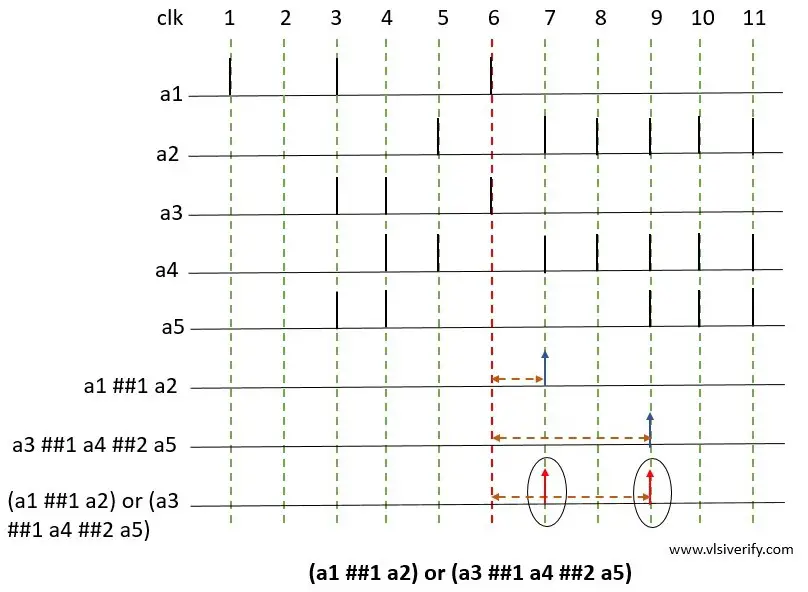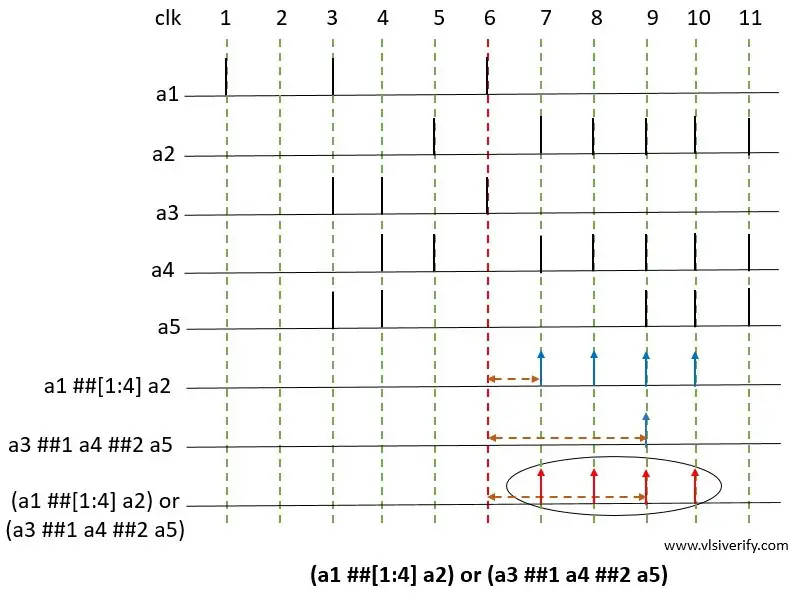Tutorials

The binary or operator is used when at least one of the two sequence operands is expected to match.

Syntax:

``<seq_exp> or <seq_exp>``

The requirement for or operation match

1. Both operand sequences should start at the same time.
2. The resultant or composite sequence match when either seq1 or seq2 matches
3. The end time of the resultant or composite sequence is the end time of the operand that finishes last.

## Example for OR of two sequences

`` (a1 ##1 a2) or (a3 ##1 a4 ##2 a5)``### Explanation

Let’s consider  a1 ##1 a2 as operand A and a3 ##1 a4 ##2 a5 as operand B.operand B

a1 ##1 a2 (Operand A): The a1 evaluates to true followed by a2 one clock tick matches at clock tick 7

a3 ##1 a4 ##2 a5 (operand B): The operand a3 evaluates to true followed by a4 one clock tick later, followed by a5 two clock ticks later i.e. at clock tick 9.

(a1 ##1 a2) and (a3 ##1 a4 ##2 a5): The resultant sequence is said to be matched when both of the above operand sequence matches. The end times of matches for individual operand sequences A and B are clock ticks 7 and 9 respectively.

The resultant sequence match should follow the conditions like individual operand sequences should start at the same clock tick (clock tick 6) and either of two operand sequences should match (clock ticks 7 and 9).

## Example for OR of two sequences including a time range

`` (a1 ##[1:4] a2) or (a3 ##1 a4 ##2 a5)``### Explanation

Let’s consider  a1 ##[1:4] a2 as operand A and a3 ##1 a4 ##2 a5 as operand B.operand B

a1 ##[1:4] a2: The first operand a1 evaluates to true followed by a2 which evaluates to true for 1,2,3, or 4 clock ticks later. So, sequence matches at clock ticks 7,8,9, and 10 respectively.

a3 ##1 a4 ##2 a5: The operand a3 evaluates to true followed by a4 one clock tick later, followed by a5 two clock ticks later i.e. at clock tick 9.

(a1 ##1 a2) and (a3 ##1 a4 ##2 a5): The resultant sequence is said to be matched when both of the above operand sequence matches. The end times of matches for individual operand sequences are clock ticks 10 and 9.

The resultant sequence match should follow the conditions like individual operand sequences should start at the same clock tick (clock tick 6) and either of two operand sequences should match (clock ticks 7,8,9 and 10).

SystemVerilog Assertions